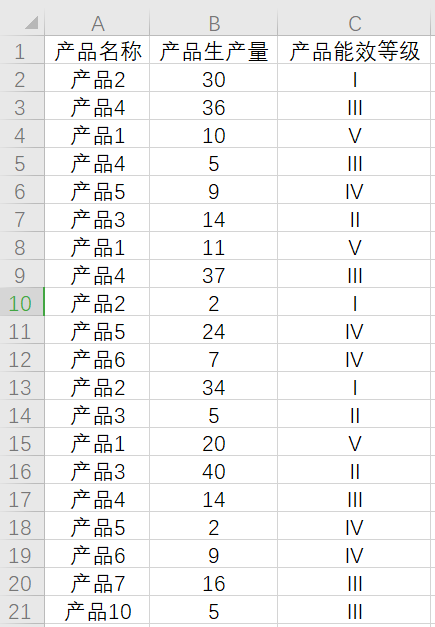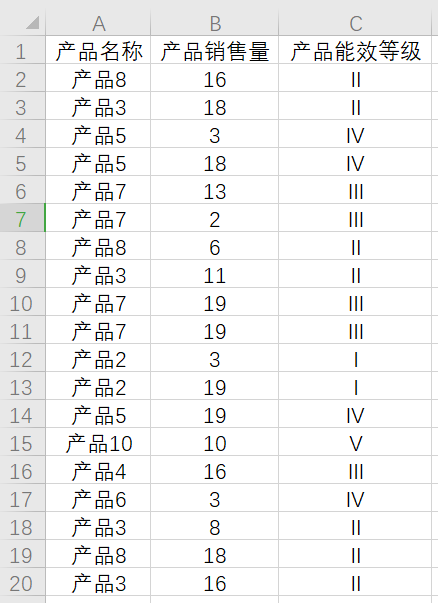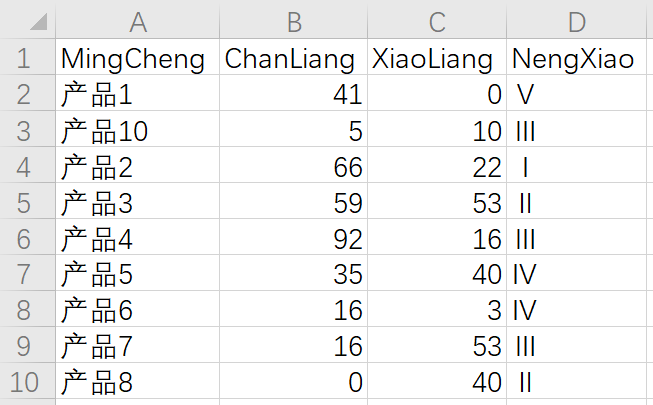• 任务描述 有两种不同类型的excel文件，每种共25个。需要将同一种类型文件汇总，并生成新的excel文件。 通过批处理语句copy *type1.xlsx all.xlsx可以实现，但有时候会...combine_list.m 合并文件程序，以函数形式...
任务描述
有两种不同类型的excel文件，每种共25个。需要将同一种类型文件汇总，并生成新的excel文件。
通过批处理语句copy *type1.xlsx all.xlsx可以实现，但有时候会乱码练习一下matlab
解决思路
两个matlab程序
combine_eyedata.m 读取文件列表；将不同类型文件分类；输出汇总后文件combine_list.m 合并文件程序，以函数形式呈现
combine_eyedata.m
clear;
input_file = 'E:\expdata\exp1\formal\eyecompute';
output_file = 'E:\expdata\exp1\formal\eyecompute_all\';

dirOutput = dir(fullfile(input_file,'*.xlsx'));
file_name_lists = {dirOutput.name};

%
converge_lists = [];
fixation_lists = [];
microsac_lists = [];
pupil_lists = [];
for i = 1:length(file_name_lists)
t = regexp(file_name_lists{i}, '_', 'split');
switch t{4}
case 'converge.xlsx'
converge_lists = [converge_lists file_name_lists(i)];
case 'fixation.xlsx'
fixation_lists = [fixation_lists file_name_lists(i)];
case 'microsac.xlsx'
microsac_lists = [microsac_lists file_name_lists(i)];
case 'pupil.xlsx'
pupil_lists = [pupil_lists file_name_lists(i)];
end
end

% converge_all = [];
% fixation_all= [];
% microsac_all = [];
% pupil_all= [];

converge_all = combine_list(input_file, converge_lists);
fixation_all = combine_list(input_file, fixation_lists);
microsac_all = combine_list(input_file, microsac_lists);
pupil_all = combine_list(input_file, pupil_lists);

xlswrite([output_file 'converge_all.xlsx'], converge_all);
xlswrite([output_file 'fixation_all.xlsx'], fixation_all);
xlswrite([output_file 'microsac_all.xlsx'], microsac_all);
xlswrite([output_file 'pupil_all.xlsx'], pupil_all);

combine_list.m
function out_list = combine_list(src, name_list)
out_list = [];
for i=1:length(name_list)
out_list = [out_list;data];
end
end

展开全文• 怎么把100多个EXCEL文件合并成一个？_EXCEL_电脑软件_编程_天涯问答_天涯社区 http://wenda.tianya.cn/question/3604d101e5cb8330
• matlabexcel里的sheet重命名废话不多说，先上代码
e = actxserver('Excel.Application');                                        % open Activex server
ewb = e.Workbooks.Open(excelPath);                                          % open file (enter full path!)

ewb.Worksheets.Item(1).Name = 'I am sheet1';                                % rename sheet 1
ewb.Worksheets.Item(2).Name = 'I am sheet2';

ewb.Worksheets.Item(1).Range('A1:J1').MergeCells=1;                         % 合并EXCEL中shett1里面A1到J1的单元格
ewb.Worksheets.Item(2).Range('A1:J1').MergeCells=1;

ewb.Save                                                                    % save to the same file
ewb.Close(false)
e.Quit

emmm代码放完，好像也没啥说的了。
为了防止你们遇到这个问题搜不到这个答案，下面多写点搜索词吧：
matlab将excel里的sheet重命名； matlab对Excel工作表重命名； 使用matlab将excel合并单元格； matlab导出excel时合并单元格；

猜你喜欢：👇🏻 ⭐【Matlab】怎么修改Excel单元格颜色？ ⭐【Matlab】你想知道在图表标题中显示变量的一切 ⭐Matlab循环读取txt文件并对其中数据进行计算最后导出为excel展开全文开发语言
• 此代码为matlab处理多批次excel的范例，可用于多个相同数据格式excel批量处理，并生成可用于论文格式的matlab曲线图
• （一）合并两个Excel的信息 描述： 假设两个表格分别为某些产品的生产量和销售量流水信息，如图， 尝试将两个表格合并，即将对应同一产品的生产量和销售量合并在同一行；且产品名称这一列，不能有重复的产品名称。...
描述： 假设两个表格分别为某些产品的生产量和销售量流水信息，如图，尝试将两个表格合并，即将对应同一产品的生产量和销售量合并在同一行；且产品名称这一列，不能有重复的产品名称。
第一步： 读取生产量、销售量数据，
[save_name,save_path] = uigetfile('*.*','选择生产量数据');

[save_name,save_path] = uigetfile('*.*','选择销售量数据');

第二步： 整理销售量数据，将产品名称相同的销售量合并到一起（第一列为产品名称、第二列为产品销售量、第三列为产品能效等级）。
class_s=unique(Data_sale{:,1});%取出互不相同的产品名称集合
n=length(class_s);

for m=1:n

[row, col] = find(cellfun(@(x) strcmp(x,class_s(m)),Data_sale{:,1}));
tmp1=Data_sale{:,2};
num_s(m)=sum(tmp1(row));%产品销售量
num_s=num_s';
tmp3=Data_sale{:,3};
stage_s(m)=tmp3(row(1));%产品能效等级
stage_s=stage_s';

end

第三步： 整理生产量数据，将产品名称相同的生产量合并到一起；将同时有生产量和销售量的产品信息合并到一起。
class_p=unique(Data_produce{:,1});%取出互不相同的产品名称集合
n=length(class_p);
for m=1:n

[row, col] = find(cellfun(@(x) strcmp(x,class_p(m)),Data_produce{:,1}));
TMP1=Data_produce{:,2};
num_p(m)=sum(TMP1(row));%产品生产量
num_p=num_p';
TMP3=Data_produce{:,3};
stage_p(m)=TMP3(row(1));%产品能效等级
[row1, col1] = find(cellfun(@(x) strcmp(x,class_p(m)),class_s));

%将生产量不为0但销售量为0的列出，销售量赋0
if sum(row1)>0
SALE(m)=num_s(row1);
else
SALE(m)=0;
end

end

第四步： 将销售量不为0但生产量为0的列出，生产量赋0，
for m=1:length(class_s)

[row2, col2] = find(cellfun(@(x) strcmp(x,class_s(m)),class_p));
if sum(row2)==0
sale_produce_class(p)=class_s(m);%产品名称
sale_produce_num(p)=num_s(m);%产品销售量
sale_produce_stage(p)=stage_s(m);%产品能效等级
p=p+1;
end

end


第五步： 合并数据输出，
MingCheng=[class_p; sale_produce_class'];
N0=length(sale_produce_num);
num_p0=zeros(N0,1);%生产量赋0
ChanLiang=[num_p;num_p0];
XiaoLiang=[SALE';sale_produce_num'];
NengXiao=[stage_p';sale_produce_stage'];

T = table(MingCheng,ChanLiang,XiaoLiang,NengXiao);
[save_name,save_path] = uiputfile('merge_excel.xlsx');%提示选择保存的路径和文件名
writetable(T,fullfile(save_path,save_name));

最终合并结果展开全文• G = importdata('g.txt');%读入文本 H = importdata('h.txt'); I = importdata('i.txt');...%读入excel out = [G;H;I;J;K]; xlswrite('z.xlsx',[out]);%将合并的结果写入z.xlsx t=out(:,1); %第 2 列为t cd=out...

G = importdata('g.txt');%读入文本 H = importdata('h.txt'); I = importdata('i.txt'); J = importdata('j.txt'); K = importdata('k.txt'); %a=xlsread('filename.xls');%读入excel out = [G;H;I;J;K]; xlswrite('z.xlsx',[out]);%将合并的结果写入z.xlsx t=out(:,1); %第 2 列为t cd=out(:,3); %第 3 列为cd cl=out(:,4); %第 3 列为cl plot(t,cd,'r');
展开全文经验分享
• matlab将多个excel读取并存到一个excel的不同sheet中
• 写入excel xlswrite(‘test.xlsx’,area,‘Result’,‘A1’)%写入字符串 xlswrite(‘test.xlsx’,heng,‘Result’,‘B1’)%写入字符串 xlswrite(‘test.xlsx’,data,‘Result’,‘B2’)%写入数据
• 1. 创建一个本地的Excel服务端,其实就是打开Excel try Excel = actxGetRunningServer('Excel.Application'); catch Excel = actxserver('Excel.Application'); end 表达意思如下 try 如果Excel 服务器已经...
• 此工具可将多个EXCEL文件进行合并，包括每个EXCEL中的每个Sheet; 还可以选择合并时在开始的两列增加日期和序号，序号是自动生成的，日期是取每个文件的文件名的第2-9位（如文件名为M20180628.xlsx ,则日期为20180626...
• Matlab代码合并Excel数据表Python数据科学入门 本入门教程将带您了解Python用于数据科学的一些工具：数学运算，统计，可视化，机器学习等。 我将假设您掌握python的知识以及该主题的一些基本知识。 我不会深入研究...
• ## matlab对Excel表读取数据

万次阅读 多人点赞 2019-07-09 23:16:25
• #MATLAB生成EXCEL文件并进行相关处理的源码 项目要求：给定某海洋站潮位数据在一年之年的每分钟潮位数据（一共有24×60×365=525600个数），txt格式，要求制作出海洋站该年份的潮汐表 实现方案：MATLAB对该txt文件...数据处理
• ## （MATLAB）多个Excel表合成一个

千次阅读 热门讨论 2020-11-27 11:54:13
把多个Excel表合成一个。手动复制粘贴太累了，就写了这个MATLAB的代码。
• matlab导入excel代码utl_import_data_from_excel_sheet_with_headers_and_footers_without_specifying_range_option 从具有标题和页脚的Excel工作表中导入数据，而无需指定RANGE选项。 关键字：sas sql join合并大...
• 本章主要讲述matlab控制Excel单元格取值，单元格字体样式选择，单元格颜色设置，单元格合并操作等
• 在处理一些实验数据时，通常要画散点图，本来EXCEL自带的散点图功能也很好，但是为了秀一下，我们也要用到MATLAB来画图，下面来介绍一下实现方法。 如果你第一次使用MATLAB，那就要从头开始介绍了。 首先来新建一个...
• matlab导入excel代码utl_quickly_read_xml_file_and_convert_to_sas_wps_dataset 快速读取xml文件并转换为SAS / WPS数据集。 关键字：sas sql join合并大数据分析宏oracle teradata mysql sas社区stackoverflow ...
• matlab导入excel代码utl_max_values_from_a_three_Dimension_array 三维数组最后一维的最大值。 关键字：sas sql join合并大数据分析宏oracle teradata mysql sas社区stackoverflow statistics人工智慧AI Python R ...
• 下面是实现这些功能的Matlab代码。我用的Matlab版本是2020版的。有些代码需要根据自己实际的需要进行更改，比如如果你读取的文件格式是.xls就可以把第五行的.csv改成.xls。或者你希望输出文件名称为别的名字，你可以...csv
• matlab导入excel代码utl_calculate_mode_for_each_row 关键词：sas sql join合并大数据分析宏oracle teradata mysql sas社区stackoverflow statistics人工智慧AI Python R Java Javascript WPS Matlab SPSS Scala ...
• 合并多个Excel文件的方法 多个文件内容简单放到一个文件里
• matlab导入excel代码utl_all_possible_pairs_of_rows 所有可能的行对。 关键字：sas sql join合并大数据分析宏oracle teradata mysql sas社区stackoverflow statistics人工智慧AI Python R Java Javascript WPS ...
• matlab合并股票代码《货币政策的债券借贷渠道》复制文件 奥利维尔·达尔穆尼，奥利弗·吉塞克，亚历山大·罗德尼扬斯基 2020 年 4 月 16 日 接触： 数据 数据是从各种公共和专有数据库中获得的。 以下枚举列出了每个...
• 生成的 Excel 文件（必须安装 Excel！）包含两个选项卡，其中包含所有文件的总体摘要：峰面积和浓度（由方法确定）。 该文件还包含原始文件名，获取的时间色谱图，文件名中的时间戳记（如下）以及所有化合物的面积/...
• 很多朋友会遇到这样的问题，就是很...下面我就介绍一种利用excel的宏计算来解决这个问题。 一、数据准备   二、合并效果 三、代码  在Sheet1上右键→查看代码，打开代码编辑器，写入如下内容： Sub UnionSheets()
•1024程序员节
• 去掉某些列 delIx = strcmp(A, 'settings') | strcmp(A, 'exposure') | strcmp(A, 'equity');... 合并去掉重复的 A= ([B, A(~ismember(A,B)) ]); 日期：1990，1,1 变成 19900101 datestr(datenum(1990,1,1),'...

# matlab合并excelmatlab 订阅